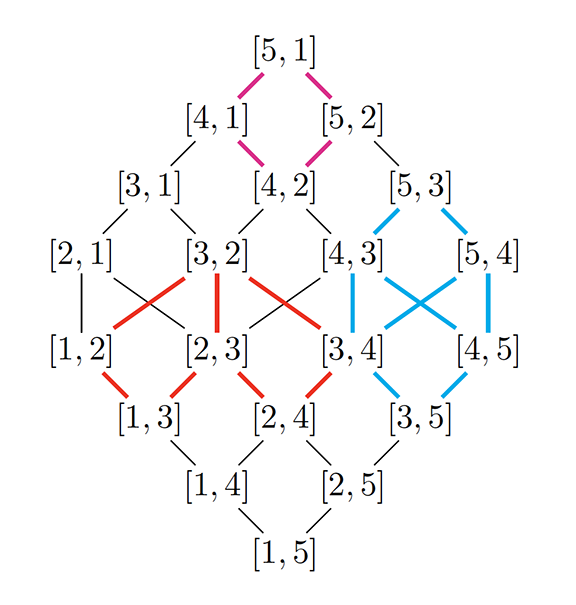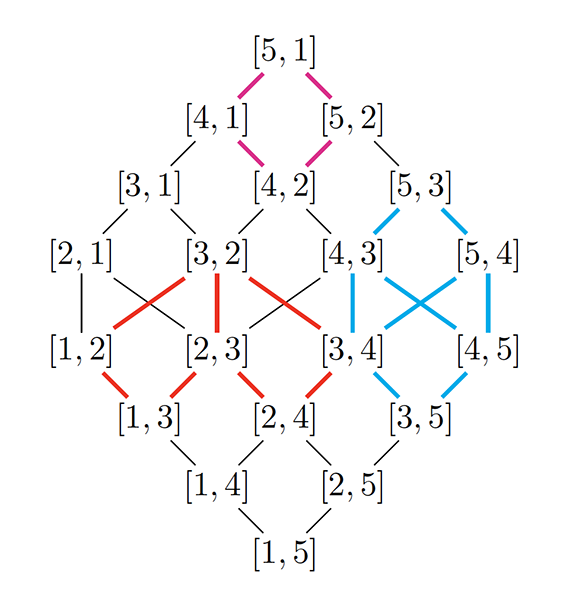### Highlights#### Connecting Configurations

ICERM - March 2022RATIONAL CURVES IN INCIDENCE VARIETIES

Consider a straight line in a plane with a point on it. Imagine moving the point on the line and moving the line in the plane. A parameter space can be constructed so that each point in the space represents a possible point-line configuration. Moreover, if one configuration can be turned into another by moving the point and the line just a little bit, then the corresponding points of the two configurations in the space are close. This space has a very nice and symmetric geometric shape. Although we cannot visualize it, we know it must "look" the same everywhere, because all the point-line configurations are created equal. This space and higher dimensional analogues of it are called incidence varieties. Geometric structures of incidence varieties are often encoded in combinatorial data such as the diagram in Figure 1.Figure 1. A diagram encoding some geometric structures in an incidence variety.Incidence varieties, and more generally, homogeneous spaces, have been studied by generations of mathematicians, but we still do not understand everything about them. One way to study these varieties is to probe them using well understood geometrical objects such as rational curves. Rational curves in a variety are classfied by a property called their degree. Maxim Konstevich, who later won the Fields medal, constructed a space called the Kontsevich moduli space, which parametrizes rational curves of a fixed degree in a given variety [Kon95]. Using the Kontsevich moduli space, information about rational curves in the variety can be organzied into a nice algebraic structure called the quantum K-theory ring [Giv00, Lee04].

During the Spring 2021 ICERM Combinatorial Algebraic Geometry semester program, Weihong Xu [Xu21] studied natural subvarieties of the Konstevich moduli space of incidence varieties and proved that they are rationally connected -- a desirable geometric property that says every two points are connected by a rational curve in the variety. This result is then used to determine the structures of the quantum K-theory rings of incidence varieties and prove some very explicit formulas that are readily available for computations.

References
[Giv00] Alexander Givental, On the wdvv equation in quantum k-theory., Michigan Mathematical Journal 48 (2000), no. 1, 295-304.
[Kon95] Maxim Kontsevich, Enumeration of rational curves via torus actions, The moduli space of curves, 1995, pp. 335-368.
[Lee04] Y-P Lee, Quantum K-theory, i: Foundations, Duke Mathematical Journal 121 (2004), no. 3, 389-424.
[Xu21] Weihong Xu, Quantum k-theory of incidence varieties, arXiv preprint arXiv:2112.13036 (2021).